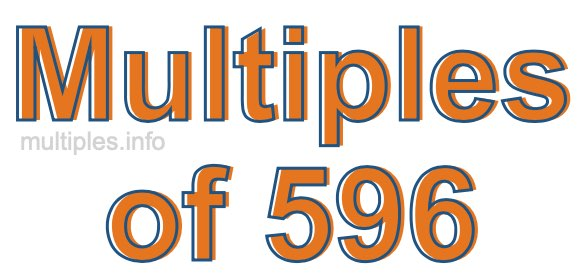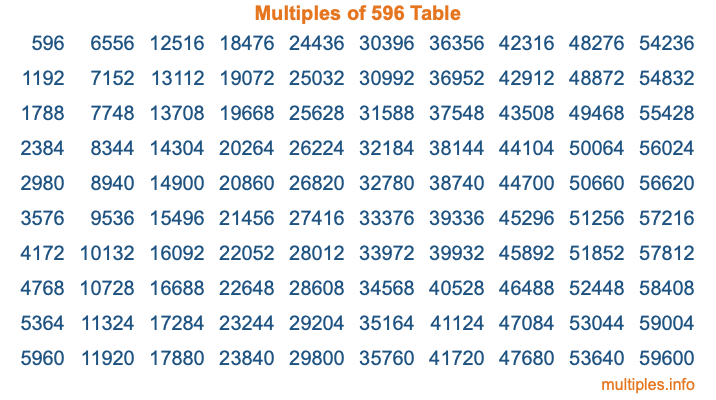Multiples of 596Welcome to the Multiples of 596 page. Here we will first teach you everything you will ever need to know about the multiples of 596, and then give you a study guide summary of everything we taught you to make sure you remember it all. Use this page to look up facts and learn information about the multiples of 596. This page will make you a multiples of five hundred ninety-six expert!

Definition of Multiples of 596
Multiples of 596 are all the numbers that when divided by 596 equal an integer. Each of the multiples of 596 are called a multiple. A multiple of 596 is created by multiplying 596 by an integer.

Therefore, to create a list of multiples of 596, you start with 1 multiplied by 596, then 2 multiplied by 596, then 3 multiplied by 596, and so on for as long as you want. Thus, the list of the first five multiples of 596 is 596, 1192, 1788, 2384, and 2980. To see a larger list of multiples of 596, see the printable image of Multiples of 596 further down on this page. We also have a category where you can choose any nth multiple of 596.

Multiples of 596 Checker
The Multiples of 596 Checker below checks to see if any number of your choice is a multiple of 596. In other words, it checks to see if there is any number (integer) that when multiplied by 596 will equal your number. To do that, we divide your number by 596. If the the quotient is an integer, then your number is a multiple of 596.

Is  a multiple of 596?

Least Common Multiple of 596 and ...
A Least Common Multiple (LCM) is the lowest multiple that two or more numbers have in common. This is also called the smallest common multiple or lowest common multiple and is useful to know when you are adding our subtracting fractions. Enter one or more numbers below (596 is already entered) to find the LCM.

Check out our LCM Calculator if you need more details about the Least Common Multiple or if you need the LCM for different numbers for adding and subtraction fractions.

nth Multiple of 596
As we stated above, 596 is the first multiple of 596, 1192 is the second multiple of 596, 1788 is the third multiple of 596, and so on. Enter a number below to find the nth multiple of 596.

th multiple of 596

Multiples of 596 vs Factors of 596
596 is a multiple of 596 and a factor of 596, but that is where the similarities end. All postive multiples of 596 are 596 or greater than 596. All positive factors of 596 are 596 or less than 596.

Below is the beginning list of multiples of 596 and the factors of 596 so you can compare:

Multiples of 596: 596, 1192, 1788, 2384, 2980, etc.

Factors of 596: 1, 2, 4, 149, 298, 596

As you can see, the multiples of 596 are all the numbers that you can divide by 596 to get a whole number. The factors of 596, on the other hand, are all the whole numbers that you can multiply by another whole number to get 596.

It's also interesting to note that if a number (x) is a factor of 596, then 596 will also be a multiple of that number (x).

Multiples of 596 vs Divisors of 596
The divisors of 596 are all the integers that 596 can be divided by evenly. Below is a list of the divisors of 596.

Divisors of 596: 1, 2, 4, 149, 298, 596

The interesting thing to note here is that if you take any multiple of 596 and divide it by a divisor of 596, you will see that the quotient is an integer.

Multiples of 596 Table
Below is an image of the first 100 multiples of 596 in a table. The table is in chronological order, column by column. The first column has the first ten multiples of 596, the second column has the next ten multiples of 596, and so on.The Multiples of 596 Table is also referred to as the 596 Times Table or Times Table of 596. You are welcome to print out our table for your studies.

Negative Multiples of 596
Although not often discussed or needed in math, it is worth mentioning that you can make a list of negative multiples of 596 by multiplying 596 by -1, then by -2, then by -3, and so on, to get the following list of negative multiples of 596:

-596, -1192, -1788, -2384, -2980, etc.

Multiples of 596 Summary
Below is a summary of important Multiples of 596 facts that we have discussed on this page. To retain the knowledge on this page, we recommend that you read through the summary and explain to yourself or a study partner why they hold true.

There are an infinite number of multiples of 596.

A multiple of 596 divided by 596 will equal a whole number.

596 divided by a factor of 596 equals a divisor of 596.

The nth multiple of 596 is n times 596.

The largest factor of 596 is equal to the first positive multiple of 596.

596 is a multiple of every factor of 596.

596 is a multiple of 596.

A multiple of 596 divided by a divisor of 596 equals an integer.

596 divided by a divisor of 596 equals a factor of 596.

Any integer times 596 will equal a multiple of 596.

Multiples of a Number
Here you can get the multiples of another number, all with the same attention to detail as we did for multiples of 596 on this page.

Multiples of
Multiples of 597
Did you find our page about multiples of five hundred ninety-six educational? Do you want more knowledge? Check out the multiples of the next number on our list!